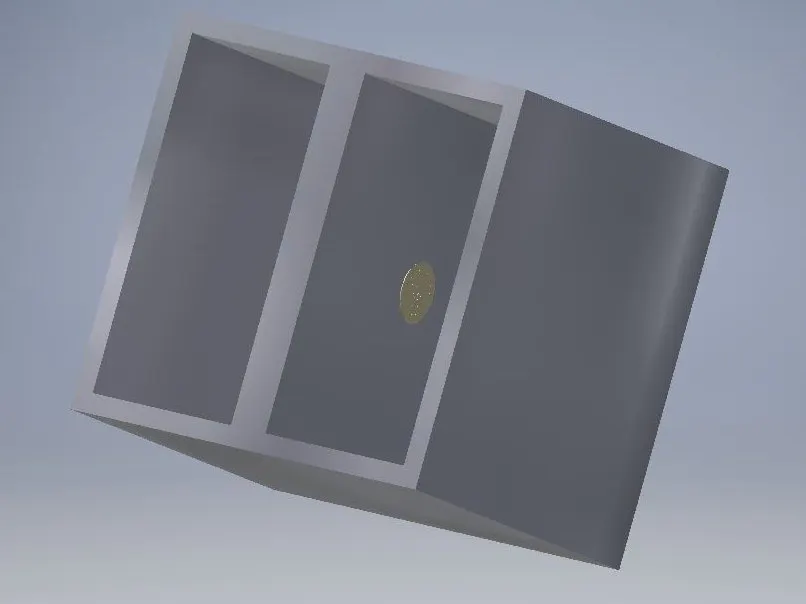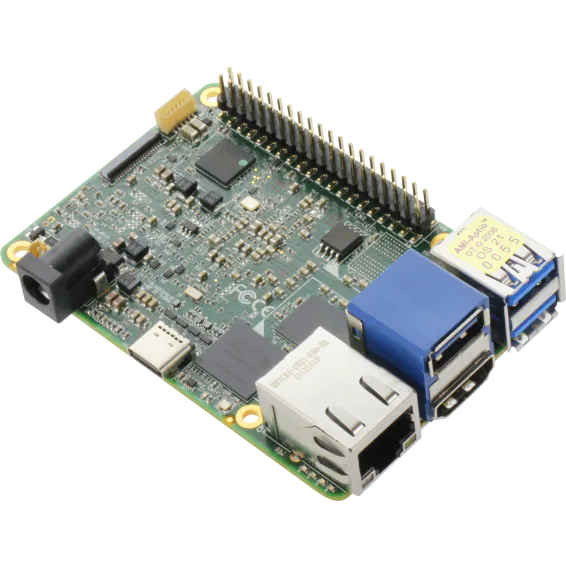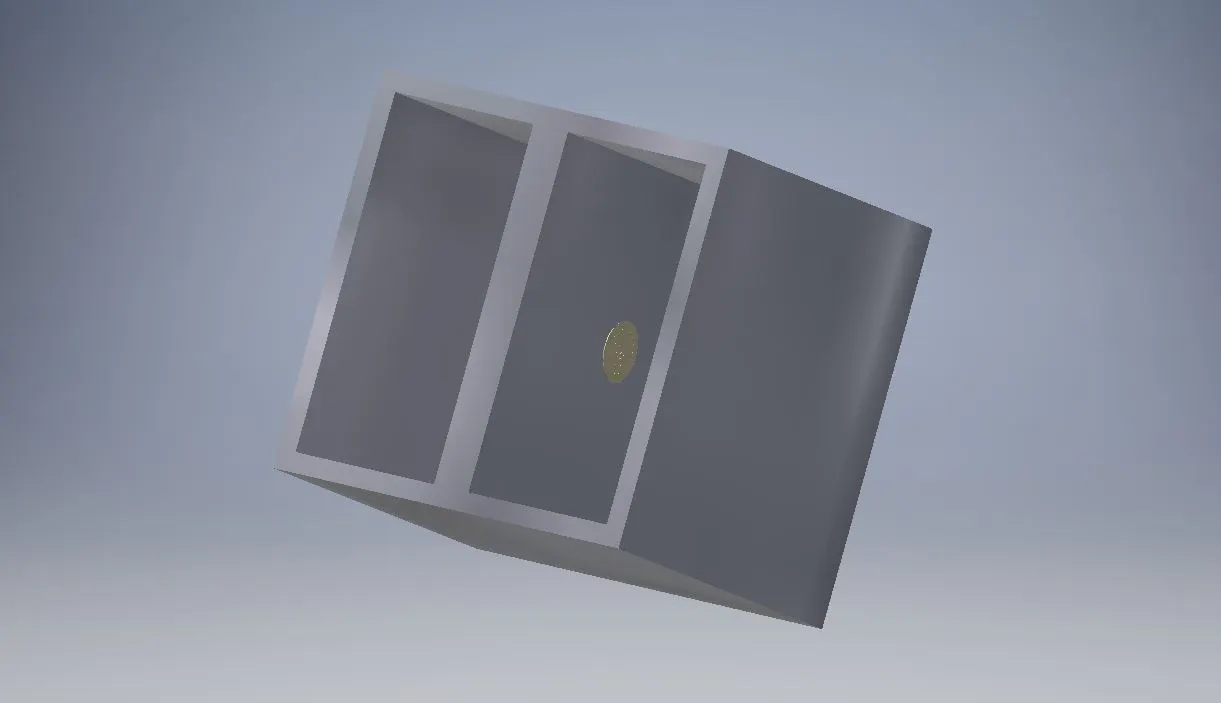Published

# Inter Alpha Inhibitor Protein (IAIP) Detector

We developed an accurate, cheap test to measure IAIP concentration in the blood and used the data to predict mortality rates of sepsis.

Showcase (no instructions)638## Things used in this project

### Hardware componentsParticle Photon
×1UP 4000 Series
×1

### Software apps and online servicesMicrosoft Azure

## Custom parts and enclosures

### Base for Galvanic Cell

The base of the galvanic cell with a porous disk.## Schematics

### IAIP Detector Diagram

The IAIP detector uses analog pin A5 to detect the voltage of the galvani cell.

## Code

C/C++
This uses a particle photon to read an analog voltage from the galvanic cell and converts these voltages with a formula to find the concentration of IAIP.
```#include <math.h>

# define EULER 2.718281828459045235360287471352
# define VCONST 0.012779

int pin = A5;
int voltRaw = 0;
double voltage = 0;
double conc = 0;
bool hasRun = false;

double simVolts = {0.008, 0.01};

void setup() {
pinMode(pin, INPUT);
pinMode(D7, OUTPUT);
}

void loop() {
//voltage = voltRaw/4095 * 3;
if(!hasRun) {
runSim();
hasRun = true;
}
}

void runSim() {
for(int i=0; i<sizeof(simVolts)/sizeof(double); i++) {
voltage = simVolts[i];
Particle.publish("Voltage:", String(voltage), 60, PRIVATE);
delay(2000);
}
conc = 0.4375 * pow(EULER, (simVolts-simVolts[sizeof(simVolts)/sizeof(double) - 1]/VCONST));
Particle.publish("IAIP Concentration:", String(conc)+" Micg/ml", 60, PRIVATE);
}
```

### Mortality Rate Finder

Java
Using IAIP data from photon, we used multivariable regression to establish a relationship between mortality rates and the prognostic factors.
```public class MortalityRateFinder {

private int SIRSval;
private double DICval;
private double MODSval;
private double IAIPval;
public MortalityRateFinder(int patientSIRS, int patientDIC, double patientMODS, double patientIAIPval) {
SIRSval = patientSIRS;
DICval = patientDIC;
MODSval = patientMODS;
IAIPval = patientIAIPval;
}
public int getSIRS() {return SIRSval;}
public double getDIC() {return DICval;}
public double getMODS() {return MODSval;}
public double getIAIP() {return IAIPval;}

public double calcMortality() {
double mortality = 2.32565811*SIRSval +  0.930263244*DICval + 0.186052649*(MODSval/100) + 6.51184271*IAIPval;
// Formula generated through parsing csv. files (n=6) on pubmed publications for data and using multivariable regression to find correlation between variables and the mortality rates. Algorithm tested on known data from other pubmed publications (n=5) with mean absolute deviation of 3.94 +/- 1.82% for mortality rates
return (int)(mortality*100)/100.0;
}
public String toString() {
if(calcMortality() <= 20) {
return "\nMortality = " + calcMortality() + "%";
}else {
return "\nMortality = " + calcMortality() +"%\nYOU ARE AT HIGH RISK OF DEATH";
}
}

}
```

### Mortality Rate Finder Runner Class

Java
Gathers input from the physician and uses Mortality Rate Finder class to calculate mortality rates.
```import java.util.*;
public class MetrohacksRun {

public static void main(String[] args) {
Scanner console = new Scanner(System.in);
int SIRS = console.nextInt();
int DIC = console.nextInt();
double MODS = console.nextDouble();
double IAIPval = console.nextDouble();

MortalityRateFinder patient = new MortalityRateFinder(SIRS, DIC, MODS, IAIPval);
System.out.println(patient);

}

}
```

## Credits

1 project • 1 follower

### Matt Sprague

1 project • 0 followers

### Leon Z

1 project • 0 followers

### Shrujal

1 project • 0 followers